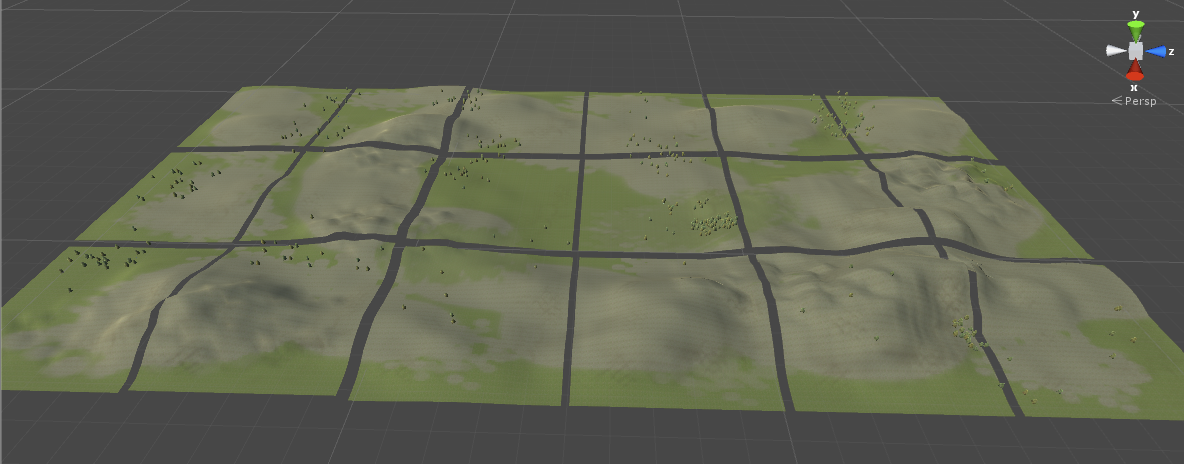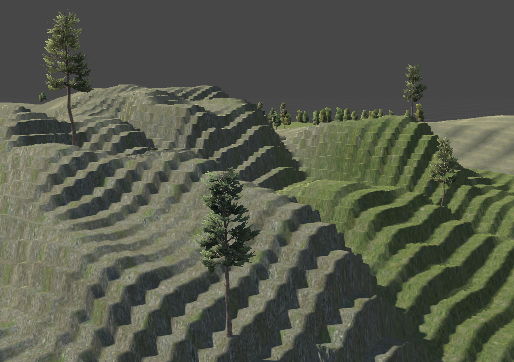## Split Unity Terrain Script

In writing this script I learned about EditorWindows: using a script to create something outside of the game running. This code will let us launch our script from the newly made menu Split -> Split Terrain. (Code must be placed in Editor directory)

```using UnityEngine;
using UnityEditor;

public class SplitTerrain : EditorWindow
{
static void Init()
{
GetWindow<SplitTerrain>();
}

public void OnGUI()
{
// Have our code here
}
}
```

When creating the Terrain Manager code, I had wished at the time I knew of a script that would take a large terrain and break it up into smaller parts. So that lead to this post. What follows is a script that takes any size terrain, allows you to enter how many pieces you want it broken into, and what you want the new heightmap, detailmap, and splatmap resolutions to be. To explain that last part, first understand what TerrainData is made of. We have the heightmap which describes the mountains and valleys. The splatmap (or alphamap) that describes what is drawn on the terrain. The detailmap describes where grass is on the terrain, and then there’s an array of tree locations. To break terrain down, we’ll need to take each of these and trim them down.Now let’s talk about sizes. A terrain’s physical size could be length 200 by width 100. The heightmap is stored in a different array of say size 513. This means that even though the terrain length is twice the size of the width, the heightmap length is 513 and the heightmap width is 513. If we cut the terrain in half lengthwise, we would be at 100 by 100, however the heightmap returned by GetHeights would be 257 by 513 now. We need to either up convert that back to 513 by 513 or go down to 257 by 257, for example. Either way we need to make up data values or lose data points. What the following code does is resize our array to any size the user specifies. heightmapResolution is the new height we are aiming for.

```// Get percent of original
float xMinNorm = xMin / origTerrain.terrainData.size.x;
float xMaxNorm = xMax / origTerrain.terrainData.size.x;
float zMinNorm = zMin / origTerrain.terrainData.size.z;
float zMaxNorm = zMax / origTerrain.terrainData.size.z;
float dimRatio1, dimRatio2;

// Height
td.heightmapResolution = heightmapResolution;
float[,] heights = origTerrain.terrainData.GetHeights(
Mathf.FloorToInt(xMinNorm * origTerrain.terrainData.heightmapWidth),
Mathf.FloorToInt(zMinNorm * origTerrain.terrainData.heightmapHeight),
Mathf.FloorToInt((xMaxNorm - xMinNorm) * origTerrain.terrainData.heightmapWidth),
Mathf.FloorToInt((zMaxNorm - zMinNorm) * origTerrain.terrainData.heightmapHeight));
float[,] newHeights = new float[heightmapResolution, heightmapResolution];
dimRatio1 = (float)heights.GetLength(0) / heightmapResolution;
dimRatio2 = (float)heights.GetLength(1) / heightmapResolution;
for (int i = 0; i < newHeights.GetLength(0); i++)
{
for (int j = 0; j < newHeights.GetLength(1); j++)
{
newHeights[i, j] = heights[Mathf.FloorToInt(i * dimRatio1), Mathf.FloorToInt(j * dimRatio2)];
}
}
td.SetHeights(0, 0, newHeights);
```

Here is the result if we upconvert back to 513. This isn’t good. Room for improvment would take and interpolate values. [Update: this has since been fixed]The trees are stored in an array the size of however many trees you have. Ten trees, size ten. Three thousand trees, size three thousand. The position is stored as a percentage of the size. If we had terrain of size 200 by 100 and one tree was dead center, it’s coord would be stored as 0.5, 0.5. If it was at the edge and to the right some, it would be 0.75, 1. Here is the code that will copy over just the trees in our new piece.

```// Tree
for (int i = 0; i < origTerrain.terrainData.treeInstanceCount; i++)
{
TreeInstance ti = origTerrain.terrainData.treeInstances[i];
if (ti.position.x < xMinNorm || ti.position.x >= xMaxNorm)
continue;
if (ti.position.z < zMinNorm || ti.position.z >= zMaxNorm)
continue;
ti.position = new Vector3(((ti.position.x * origTerrain.terrainData.size.x) - xMin) / (xMax - xMin), ti.position.y, ((ti.position.z * origTerrain.terrainData.size.z) - zMin) / (zMax - zMin));
}
```

To explain getting the new position code, if our tree was originally at 0.8 and we were cutting our terrain from 200 to 100 (and keeping the later half), we do 0.8 * 200 which is 160, then subtract the removed part of 100, then divide by 200 minus 100. The new position is 0.6.

You also need to create an asset and save it. Like this.

```if (!AssetDatabase.IsValidFolder("Assets/Resources"))
AssetDatabase.CreateFolder("Assets", "Resources");
// Must do this before Splat
AssetDatabase.CreateAsset(td, "Assets/Resources/" + newName + ".asset");

// Make our terrain

AssetDatabase.SaveAssets();
```

I save to Resources so I can load it using Resources.LoadAsync in the Terrain Manager code.

If you know of a way to improve this, please let me know. Note the GitHub code is actually at a state where I’ve split some terrain already and you can hit Play and walk around. Here is the link for SplitTerrain.cs.

This code was based on code by Kostiantyn Dvornik. Much thanks to him for sharing!